Car

Car is going 95 km per hour. How long distance goes in 1 minute?

Result

l =  1583.3 m

Solution:Leave us a comment of this math problem and its solution (i.e. if it is still somewhat unclear...):Be the first to comment!To solve this verbal math problem are needed these knowledge from mathematics:

Do you want to convert length units?

Next similar math problems:

1. Train and walker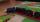Walker passes an hour 5 km. Express train per hour 100 km. Walker went 4 hours. How many minutes took the distance express train?
2. Avg speed of flight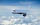The students vice adventure had a 2,367 km flight. If they travel time was 2 hours and 56 minutes, what was their average speed in kilometres per hour?
3. Cyclist vs car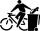Cyclist rode out of the city at 18 km/h. 1 hour 30 minutes behind him started car and caught up with the cyclist in 50 minutes. How fast was driving the car? Where (what kilometer) from the city car overtook a cyclist?
4. Hr to minSue biked to school in 5/12 of an hour. How many minutes did it take her to ride to school?
5. Cyclist 9A cyclist travels at a a speed of 4.25 km per hour. At that rate, how far can he travel in 3.75 hours?
6. WalkersWalker, which makes 120 steps per minute, make distance from point A to point B for 55 minutes. The length of his step is 75cm. For how long does this distance go boy who will do 110 steps 60 cm long in a minute?
7. Two cars 2Two cars started from two positions 87 km distant at the same time in opposite directions at speeds 81 km/h and 75 km/h. What was the distance between them after 2 hours 50 minutes of driving.
8. Geometric plan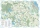At what scale the building plan if one side of the building is 45m long and 12mm long on a plan?
9. Knight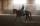Knight passed 13 km long track in 26 minutes, what was his average speed?
10. Aircraft nose downHow long will fall airliner from a height of 10000 m at speed 1,000 km/h?
11. HoursThe lesson lasts 45 minutes. For the week, students have 18 lesson hours. How many are the actual hours?
12. Average speedMichal went out of the house by car at speed 98 km/h. He came into the goal place for 270 minutes. Determine the distance between the two places.
13. Speeds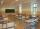George pass on the way to school distance 200 meters in 165 seconds. What is the average walking speed in m/s and km/h?
14. Pedestrian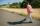Dana started at 10:00 from the point A to point B. These points are distant 12 km. Determine how fast Daniel went when the place B arrived at 11:54. Speed express in km/h.
15. Bus 14Boatesville is 65.35 kilometers from Stanton. A bus traveling from Stanton is 24.13 kilometers from Boatesville. How far has the bus traveled?
16. WavelengthCalculate the wavelength of the tone frequency 11 kHz if the sound travels at speeds of 343 m/s.
17. Fan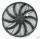The fan has a speed of 210 RPM. Calculate for time of one fan period.